# JP5463265B2 - Wide angle lens - Google Patents

## Info

Publication number
JP5463265B2
JP5463265B2 JP2010256885A JP2010256885A JP5463265B2 JP 5463265 B2 JP5463265 B2 JP 5463265B2 JP 2010256885 A JP2010256885 A JP 2010256885A JP 2010256885 A JP2010256885 A JP 2010256885A JP 5463265 B2 JP5463265 B2 JP 5463265B2
Authority
JP
Japan
Prior art keywords
lens
wide
refractive power
angle
image
Prior art date
Legal status (The legal status is an assumption and is not a legal conclusion. Google has not performed a legal analysis and makes no representation as to the accuracy of the status listed.)
Expired - Fee Related
Application number
JP2010256885A
Other languages
Japanese (ja)
Other versions
JP2012108302A (en
Inventor

Original Assignee

Priority date (The priority date is an assumption and is not a legal conclusion. Google has not performed a legal analysis and makes no representation as to the accuracy of the date listed.)
Filing date
Publication date
Application filed by 株式会社タムロン filed Critical 株式会社タムロン
Priority to JP2010256885A priority Critical patent/JP5463265B2/en
Publication of JP2012108302A publication Critical patent/JP2012108302A/en
Application granted granted Critical
Publication of JP5463265B2 publication Critical patent/JP5463265B2/en
Expired - Fee Related legal-status Critical Current
Anticipated expiration legal-status Critical

• 230000014509 gene expression Effects 0.000 claims description 16
• 230000004075 alteration Effects 0.000 description 55
• 238000003384 imaging method Methods 0.000 description 32
• 238000010586 diagrams Methods 0.000 description 24
• 206010010071 Coma Diseases 0.000 description 21
• 230000002093 peripheral Effects 0.000 description 15
• 230000003287 optical Effects 0.000 description 13
• 239000006059 cover glasses Substances 0.000 description 7
• 230000001629 suppression Effects 0.000 description 4
• 230000035945 sensitivity Effects 0.000 description 2
• 210000003733 Optic Disk Anatomy 0.000 description 1
• 206010073261 Ovarian theca cell tumour Diseases 0.000 description 1
• 208000001644 Thecoma Diseases 0.000 description 1
• 230000000295 complement Effects 0.000 description 1
• 230000000694 effects Effects 0.000 description 1
• 229910044991 metal oxides Inorganic materials 0.000 description 1
• 150000004706 metal oxides Chemical class 0.000 description 1
• 239000004065 semiconductors Substances 0.000 description 1

## Images

•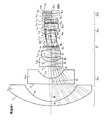•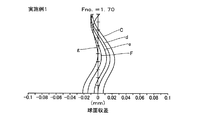•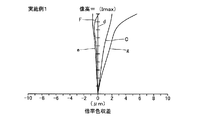•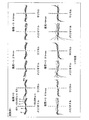•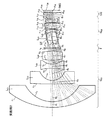•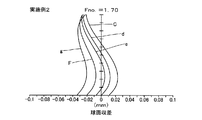•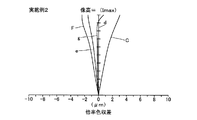•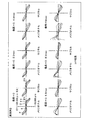•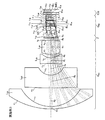•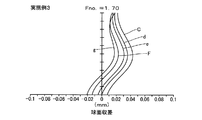•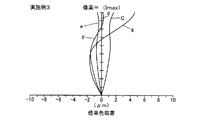•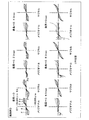## Classifications

• GPHYSICS
• G02OPTICS
• G02BOPTICAL ELEMENTS, SYSTEMS, OR APPARATUS
• G02B13/00Optical objectives specially designed for the purposes specified below
• G02B13/18Optical objectives specially designed for the purposes specified below with lenses having one or more non-spherical faces, e.g. for reducing geometrical aberration
• GPHYSICS
• G02OPTICS
• G02BOPTICAL ELEMENTS, SYSTEMS, OR APPARATUS
• G02B13/00Optical objectives specially designed for the purposes specified below
• G02B13/06Panoramic objectives; So-called "sky lenses" including panoramic objectives having reflecting surfaces
• GPHYSICS
• G02OPTICS
• G02BOPTICAL ELEMENTS, SYSTEMS, OR APPARATUS
• G02B9/00Optical objectives characterised both by the number of the components and their arrangements according to their sign, i.e. + or -
• G02B9/34Optical objectives characterised both by the number of the components and their arrangements according to their sign, i.e. + or - having four components only

## Description

The present invention relates to a wide-angle lens suitable for an electronic imaging apparatus provided with an imaging element such as a CCD (Charged Coupled Device) or a CMOS (Complementary Metal Oxide Semiconductor).

Conventionally, as an imaging lens for surveillance cameras, a wide-angle lens having a front group composed of a plurality of lenses and a rear group composed of a plurality of lenses from the object side to the image side with a stop as a boundary Is known (see, for example, Patent Document 1).

The wide-angle lens disclosed in Patent Document 1 includes a negative front group, an aperture stop, and a positive rear group in order from the object side, and the front group has a concave surface directed toward the image side in order from the object side. The rear group includes four negative lenses, three positive lenses and one positive lens, and the rear group includes a single positive lens closest to the image side.

JP 2004-102162 A

The imaging lens used in the surveillance camera must have a high imaging performance over the entire effective screen while being wide-angled in order to ensure a good field of view over a wide range so as not to cause blind spots. Desired. Further, since the surveillance camera is used at night, it is also required that the imaging lens for the surveillance camera is a bright lens. In addition, it is also required to increase the chief ray incident angle to the peripheral image height image pickup element and suppress the decrease in peripheral light amount by reducing the size of the image pickup apparatus. However, there are no conventional imaging lenses such as the wide-angle lens described in Patent Document 1 that satisfy this requirement.

For example, since the wide-angle lens described in Patent Document 1 has an angle of view of about 170 degrees, the condition of wide angle is satisfied. However, since the wide-angle lens described in Patent Document 1 has insufficient correction of lateral chromatic aberration, it cannot be said to have high imaging performance. Furthermore, since the wide-angle lens described in Patent Document 1 has an F value of 2.3, sufficient brightness cannot be ensured particularly when used at night, which is not preferable as an imaging lens for a surveillance camera.

An object of the present invention is to provide a small-sized wide-angle lens having high imaging performance over the entire effective screen while being bright and wide-angle in order to solve the above-described problems caused by the conventional technology. .

In order to solve the above-described problems and achieve the object, a wide-angle lens according to the invention of claim 1 includes a first lens group having a negative refractive power, an aperture stop, and a positive aperture arranged in order from the object side. A first lens group having a negative refractive power with a convex surface facing the object side and a concave surface facing the image side in order from the object side. A second lens having a negative refractive power, a third lens having a negative refractive power, and a fourth lens having a positive refractive power are arranged, and the second lens group is formed from the object side. Sequentially, a cemented lens including a fifth lens and a sixth lens and having an overall positive refractive power, a seventh lens having a positive refractive power, and an eighth lens having a negative refractive power with a concave surface facing the image side. And a lens.

According to the first aspect of the present invention, it is possible to satisfactorily correct various aberrations including lateral chromatic aberration and coma.

In a wide-angle lens according to the invention of claim 2 is the invention according to claim 1, characterized by satisfying the following conditional expression.
(1) AB1 <AB2
(2) AB1 <45
Here, AB1 represents the Abbe number with respect to the d-line of the lens arranged closest to the image side in the wide-angle lens, and AB2 represents the Abbe number with respect to the d-line of the lens arranged second most from the image side in the wide-angle lens.

According to the second aspect of the present invention, it is possible to suppress the occurrence of lateral chromatic aberration and coma aberration at the peripheral image height.

According to the present invention, there is an effect that it is possible to provide a small-sized wide-angle lens that is bright and wide-angle but has high imaging performance over the entire effective screen.

1 is a cross-sectional view along the optical axis showing the configuration of a wide-angle lens according to Example 1. FIG. FIG. 3 is a spherical aberration diagram of the wide-angle lens according to Example 1; FIG. 3 is a chromatic aberration diagram of a wide angle lens according to Example 1; FIG. 3 is a coma aberration diagram of the wide-angle lens according to Example 1; 6 is a cross-sectional view along the optical axis showing the configuration of a wide-angle lens according to Example 2. FIG. 6 is a spherical aberration diagram of the wide-angle lens according to Example 2. FIG. FIG. 6 is a chromatic aberration diagram of a wide angle lens according to Example 2; FIG. 6 is a coma aberration diagram of the wide-angle lens according to Example 2; 6 is a cross-sectional view along the optical axis showing the configuration of a wide-angle lens according to Example 3. FIG. 6 is a spherical aberration diagram of the wide-angle lens according to Example 3. FIG. FIG. 6 is a chromatic aberration diagram of a wide-angle lens magnification according to Example 3; FIG. 9 is a coma aberration diagram of the wide-angle lens according to Example 3;

Hereinafter, preferred embodiments of the wide-angle lens according to the present invention will be described in detail.

The wide-angle lens according to the present invention includes a first lens group having a negative refractive power, an aperture stop, and a second lens group having a positive refractive power, which are arranged in order from the object side. The first lens group is composed of four or more lenses, the second lens group is composed of four or more lenses, and a negative lens is disposed closest to the image side of the second lens group. Has been. With this configuration, it is possible to satisfactorily correct lateral chromatic aberration and coma. In particular, when this wide-angle lens is composed of eight or more lenses, the coma aberration and peripheral image height that were caused by the curvature radius of the surface becoming smaller with seven or less lenses could not be corrected. Generation of lateral chromatic aberration can be effectively suppressed.

Further, by causing the concave surface of the negative lens disposed closest to the image side of the second lens group to face the image side, the vignetting of the light beam at the peripheral image height is reduced, and the reduction of the peripheral light amount can be suppressed. Further, when the positive lens is arranged second most from the image side of the second lens group, the negative chromatic aberration and coma aberration are canceled together with the negative lens arranged closest to the image side, and the chromatic aberration of magnification and coma are corrected. Aberration can be more effectively suppressed.

In view of the above characteristics, in consideration of more effective correction of other aberrations (for example, spherical aberration), the wide-angle lens according to the present invention may be configured as follows. For example, a first lens group having a negative refractive power, an aperture stop, and a second lens group having a positive refractive power, which are sequentially arranged from the object side, are provided, and the first lens group is disposed on the object side. In order, a first lens having negative refractive power with a convex surface facing the object side, a second lens having negative refractive power with a concave surface facing the image side, and a third lens having negative refractive power, And a fourth lens having a positive refractive power, and the second lens group is composed of a fifth lens and a sixth lens in order from the object side, and has a positive refractive power as a whole. And a seventh lens having a positive refractive power and an eighth lens having a negative refractive power with the concave surface facing the image side may be arranged. Thus, by including a cemented lens, various aberrations such as longitudinal chromatic aberration as well as lateral chromatic aberration and coma aberration can be corrected well. Furthermore, by forming an aspherical surface on the second lens or the eighth lens, it is possible to expect better correction of spherical aberration and coma aberration.

An object of the present invention is to provide a small wide-angle lens that is bright and wide-angle and has high imaging performance over the entire effective screen so that it can be used for a surveillance camera. Therefore, in order to achieve this purpose, various conditions as shown below are set.

First, in the wide-angle lens according to the present invention, when the Abbe number with respect to the d-line of the lens arranged closest to the image side is AB1, and the Abbe number with respect to the d-line of the lens arranged second most from the image side is AB2, It is preferable that the following conditional expression is satisfied.
(1) AB1 <AB2
(2) AB1 <45

As described above, the Abbe number with respect to the d-line of the lens arranged second most from the image side is made smaller than the Abbe number with respect to the d-line of the lens arranged second most from the image side. It is possible to effectively suppress the occurrence of lateral chromatic aberration and coma aberration at the peripheral image height, which is a problem with the lens. Note that when the lens arranged closest to the image side has an Abbe number of 45 or more with respect to the d-line, the occurrence of lateral chromatic aberration and coma aberration at the peripheral image height cannot be suppressed.

In the wide-angle lens according to the present invention, the following configuration can also be adopted in order to suppress the occurrence of lateral chromatic aberration and coma aberration at the peripheral image height. That is, a first lens group having a negative refractive power, an aperture stop, and a second lens group having a positive refractive power, which are arranged in order from the object side, include four or more first lens groups. The second lens group is composed of four or more lenses, and an aspherical surface satisfying the following conditional expression is formed on the image side surface of the lens disposed closest to the image side of the second lens group. Form.
(3) X (0.7H)> 0
Where X is the amount of aspherical sag from the top of the optical axis surface (the direction of the image plane is positive), H is the distance from the optical axis to the lens outer diameter direction, R is the paraxial radius of curvature, and ε is the cone coefficient , A, B, C, D, E, F, G, I, J, K, L, M are secondary, tertiary, quaternary, fifth, sixth, seventh, eighth, ninth, respectively. , 10th order, 11th order, 12th order, 13th order aspherical coefficient, X (0.7H) is from the center of the optical axis with respect to the effective diameter of the image side surface of the lens disposed closest to the image side of the second lens group. The aspherical shape sag amount at a position 70% of the distance H is shown.

The reason for considering the amount of aspherical sag at 70% of the distance H from the center of the optical axis with respect to the effective diameter of the image side surface of the lens disposed closest to the image side in the second lens group is the second reason. This is because the light beam passing through the lens disposed at the most image side of the lens group exhibits high light collection efficiency in the image sensor.

By the way, an image sensor such as a CCD or CMOS has a lower sensitivity to obliquely incident light than a silver salt film, so that a chief ray angle (CRA) is an incident angle of a light beam incident on the image sensor. Is required to be small. On the other hand, in recent years, there has been a demand for further downsizing of the image pickup apparatus, so that it is required to shorten the total length of the optical system, and it is also necessary to improve the sensitivity of the image pickup element with respect to incident light from an oblique direction. Thus, in recent years, image sensors having a recommended CRA of the peripheral image height of about 15 to 30 degrees have become mainstream. Further, on the light receiving surface (imaging plane) of the image sensor, the position at which the image height is 70% of the maximum image height has the highest light collection efficiency.

Therefore, in the wide-angle lens according to the present invention, the incident angle of the principal ray of the light beam that forms an image at a position that is 70% image height with respect to the maximum image height I max is θ (0.7I max ). It is preferable that the following conditional expression is satisfied.
(4) 15 <θ (0.7I max ) <30

By satisfying the conditional expression (4), it is possible to reduce the vignetting of the light beam formed at the peripheral image height and to suppress the decrease in the peripheral light amount. Here, if the upper limit is exceeded or less than the lower limit in the conditional expression (4), vignetting occurs in a part of the light beam formed at the peripheral image height, leading to a decrease in the peripheral light amount.

As described above, the wide-angle lens according to the present invention has the above-described characteristics, so that high imaging performance can be obtained over the entire effective screen while being bright and wide-angle. That is, by providing the above features, a small wide-angle lens suitable for a surveillance camera, for example, an F value of about 1.7 and an angle of view of 200 degrees or more, while effectively correcting chromatic aberration up to the image periphery. A small wide-angle lens having excellent imaging performance that can be obtained is obtained.

Hereinafter, embodiments of a wide-angle lens according to the present invention will be described in detail with reference to the drawings. The present invention is not limited to the following examples.

FIG. 1 is a cross-sectional view along the optical axis showing the configuration of the wide-angle lens according to the first example. This wide-angle lens includes, in order from the object side (not shown), a first lens group G 11 having a negative refractive power, an aperture stop ST that defines a predetermined aperture, and a second lens group G 12 having a positive refractive power. Are arranged. Further, in this wide-angle lens, between the first lens group G 11 and the aperture stop ST, IR cut filter F is arranged to cut off the infrared, between the second lens group G 12 and the image plane IMG Is provided with a cover glass CG. The IR cut filter F and the cover glass CG are arranged as necessary and can be omitted. Note that a light receiving surface of an image sensor such as a CCD or a CMOS is disposed on the imaging plane IMG.

The first lens group G 11 has, in order from the object side, a first lens L 11 having a negative refractive power with a convex surface facing the object side, and a negative refractive power with a concave surface facing the imaging plane IMG side. A second lens L 12 having a negative refractive power, a third lens L 13 having a negative refractive power, and a fourth lens L 14 having a positive refractive power are arranged. Further, on both sides of the second lens L 12, aspheric surface is formed.

The second lens group G 12 includes, sequentially from the object side, the seventh lens L having a cemented lens having a positive refractive power as a whole composed of a fifth lens L 15 sixth lens L 16 Prefecture, the positive refractive power 17 and an eighth lens L 18 having a negative refractive power with the concave surface facing the imaging plane IMG are arranged. An aperture stop ST is provided on the object side surface of the fifth lens L 15 . In addition, aspheric surfaces are formed on both surfaces of the seventh lens L 17 and the eighth lens L 18 .

Various numerical data related to the wide-angle lens according to Example 1 are shown below.

Focal length of entire wide-angle lens = 1.10 (mm)
F value = 1.70
Maximum angle of view = 208 °
Maximum image height (I max ) = (2.0mm)

(Numerical values related to conditional expressions (1) and (2))
AB1 = 25.6, AB2 = 56.0
(AB1 <AB2, AB1 <45)

(Numerical values related to conditional expression (3))
X (0.7H) = 0.176 (> 0)
Effective diameter of the eighth lens L 18 : φ2.9

(Numerical values related to conditional expression (4))
(15 <) θ (0.7I max ) = 19.4 ° (<30)

r 1 = 15.9327
d 1 = 0.9971 nd 1 = 1.8830 νd 1 = 40.8
r 2 = 6.9238
d 2 = 3.9810
r 3 = 146.0847 (aspherical surface)
d 3 = 1.3388 nd 2 = 1.5312 νd 2 = 56.0
r 4 = 3.2097 (aspherical surface)
d 4 = 2.7843
r 5 = -17.3963
d 5 = 1.0190 nd 3 = 1.4875 νd 3 = 70.2
r 6 = 3.0841
d 6 = 0.9921
r 7 = 10.8096
d 7 = 1.8017 nd 4 = 2.0007 νd 4 = 25.5
r 8 = -9.6129
d 8 = 0.5500
r 9 = ∞
d 9 = 0.2500 nd 5 = 1.5163 νd 5 = 64.1
r 10 = ∞
d 10 = 1.5866
r 11 = 4.5388
d 11 = 1.4277 nd 6 = 1.4970 νd 6 = 81.5
r 12 = -2.1860
d 12 = 0.6605 nd 7 = 1.9459 νd 7 = 18.0
r 13 = -3.3032
d 13 = 0.1000
r 14 = 4.7836 (aspherical surface)
d 14 = 1.5165 nd 8 = 1.5312 νd 8 = 56.0
r 15 = -3.5486 (aspherical surface)
d 15 = 0.1000
r 16 = -17.1750 (aspherical surface)
d 16 = 0.6871 nd 9 = 1.6142 νd 9 = 25.6
r 17 = 2.9857 (aspherical surface)
d 17 = 1.000
r 18 = ∞
d 18 = 0.5000 nd 10 = 1.5163 νd 10 = 64.1
r 19 = ∞
d 19 = 0.5050
r 20 = ∞ (imaging plane)

Cone coefficient (ε) and aspheric coefficient (A, B, C, D, E, F, G, I, J, K, L, M)
(Third side)
ε = -26.7977,
A = 0, B = 0,
C = 1.6735 × 10 −4 , D = 0
E = -1.4609 × 10 -5 , F = 0,
G = 3.2201 × 10 -7 , I = 0
J = -2.2695 × 10 -9 , K = 0,
L = 0, M = 0
(Fourth surface)
ε = -3.4148,
A = 0, B = 0,
C = 1.5835 × 10 −2 , D = 0
E = -1.1387 × 10 -3 , F = 0,
G = 1.3770 × 10 −4 , I = 0,
J = -7.4376 × 10 -6 , K = 0,
L = 0, M = 0
(14th page)
ε = -42.2987,
A = 0, B = 1.4812 × 10 -2 ,
C = 2.5477 × 10 −2 , D = −2.2023 × 10 −3 ,
E = -1.2630 × 10 -2 , F = -1.5868 × 10 -4 ,
G = 6.1562 × 10 −3 , I = -1.4662 × 10 −3 ,
J = -9.4876 × 10 −4 , K = 4.9961 × 10 −5 ,
L = 3.3281 × 10 −4 , M = −9.3351 × 10 −5
(15th page)
ε = -0.9944,
A = 0, B = 3.7469 × 10 -2 ,
C = -1.1746 × 10 −2 , D = 4.3306 × 10 −3 ,
E = 2.4489 × 10 -4 , F = -1.3142 × 10 -3 ,
G = -7.9739 × 10 −4 , I = −8.0732 × 10 −5 ,
J = 2.4372 × 10 −4 , K = 2.1606 × 10 −4 ,
L = 2.6246 × 10 -5 , M = -6.2389 × 10 -5
(16th surface)
ε = 60.7114,
A = 0, B = 7.66637 × 10 −3 ,
C = 7.8958 × 10 −3 , D = -1.1692 × 10 −2 ,
E = -2.7694 × 10 -3 , F = 6.3880 × 10 -4 ,
G = 8.5334 × 10 −4 , I = 8.2667 × 10 −3 ,
J = -2.4691 × 10 −3 , K = −8.6688 × 10 −3 ,
L = 7.0636 × 10 -3 , M = -1.5798 × 10 -3
(Seventeenth surface)
ε = -7.8466,
A = 0, B = 2.8982 × 10 −2 ,
C = -3.2293 × 10 -2 , D = 4.3727 × 10 -2 ,
E = -1.1298 × 10 -2 , F = -1.9521 × 10 -3 ,
G = 2.1547 × 10 −3 , I = 3.7162 × 10 −4 ,
J = -2.8823 × 10 -4 , K = 1.0327 × 10 -4 ,
L = 8.7945 × 10 −5 , M = −6.6464 × 10 −5

FIG. 2 is a spherical aberration diagram of the wide-angle lens according to Example 1. FIG. 3 is a diagram of chromatic aberration of magnification of the wide-angle lens according to the first example. FIG. 4 is a coma aberration diagram of the wide-angle lens according to Example 1. In the figure, d is d line (λ = 588 nm), g is g line (λ = 436 nm), F is F line (λ = 486 nm), C is C line (λ = 656 nm), e is e line (λ = 546 nm). Further, I max in the lateral chromatic aberration diagram and coma aberration diagram represents the maximum image height.

FIG. 5 is a cross-sectional view along the optical axis showing the configuration of the wide-angle lens according to the second example. This wide-angle lens includes, in order from the object side (not shown), a first lens group G 21 having a negative refractive power, an aperture stop ST that defines a predetermined aperture, and a second lens group G 22 having a positive refractive power. Are arranged. In this wide-angle lens, an IR cut filter F that blocks infrared rays is disposed between the first lens group G 21 and the aperture stop ST, and between the second lens group G 22 and the imaging plane IMG. Is provided with a cover glass CG. The IR cut filter F and the cover glass CG are arranged as necessary and can be omitted. Note that a light receiving surface of an image sensor such as a CCD or a CMOS is disposed on the imaging plane IMG.

The first lens group G 21 has, in order from the object side, a first lens L 21 having a negative refractive power with a convex surface facing the object side, and a negative refractive power with a concave surface facing the imaging surface IMG side. The second lens L 22 having a negative refractive power, the third lens L 23 having a negative refractive power, and the fourth lens L 24 having a positive refractive power are arranged. Further, on both sides of the second lens L 22, aspheric surface is formed.

The second lens group G 22 includes, in order from the object side, a cemented lens including a fifth lens L 25 and a sixth lens L 26 and having a positive refractive power as a whole, and a seventh lens L having a positive refractive power. 27 and an eighth lens L 28 having a negative refractive power with a concave surface facing the imaging plane IMG are arranged. An aperture stop ST is provided on the object side surface of the fifth lens L 25 . Further, on both surfaces of the eighth lens L 28, aspheric surface is formed.

Various numerical data relating to the wide-angle lens according to Example 2 will be described below.

Focal length of entire wide-angle lens = 1.10 (mm)
F value = 1.70
Maximum angle of view = 206 °
Maximum image height (I max ) = (2.0mm)

(Numerical values related to conditional expressions (1) and (2))
AB1 = 40.7, AB2 = 61.1
(AB1 <AB2, AB1 <45)

(Numerical values related to conditional expression (3))
X (0.7H) = 0.155 (> 0)
Effective diameter of the eighth lens L 28 : φ2.9

(Numerical values related to conditional expression (4))
(15 <) θ (0.7I max ) = 19.2 ° (<30)

r 1 = 16.0864
d 1 = 1.2907 nd 1 = 1.8830 νd 1 = 40.8
r 2 = 6.9416
d 2 = 3.9838
r 3 = 113.0652 (aspherical surface)
d 3 = 0.7846 nd 2 = 1.5312 νd 2 = 56.0
r 4 = 2.8449 (aspherical surface)
d 4 = 2.7591
r 5 = -12.5199
d 5 = 0.9430 nd 3 = 1.4875 νd 3 = 70.2
r 6 = 3.4817
d 6 = 0.8955
r 7 = 10.2345
d 7 = 2.0614 nd 4 = 2.0007 νd 4 = 25.5
r 8 = -10.4381
d 8 = 0.5500
r 9 = ∞
d 9 = 0.2500 nd 5 = 1.5163 νd 5 = 64.1
r 10 = ∞
d 10 = 1.5866
r 11 = 4.2276
d 11 = 1.9169 nd 6 = 1.4970 νd 6 = 81.5
r 12 = -2.1540
d 12 = 0.6141 nd 7 = 1.9459 νd 7 = 18.0
r 13 = -3.3389
d 13 = 0.1000
r 14 = 3.5849
d 14 = 1.3275 nd 8 = 1.5891 νd 8 = 61.1
r 15 = -8.1241
d 15 = 0.1000
r 16 = -51.7825 (aspherical surface)
d 16 = 0.6980 nd 9 = 1.8061 νd 9 = 40.7
r 17 = 3.8023 (aspherical surface)
d 17 = 1.000
r 18 = ∞
d 18 = 0.5000 nd 10 = 1.5163 νd 10 = 64.1
r 19 = ∞
d 19 = 0.5043
r 20 = ∞ (imaging plane)

Cone coefficient (ε) and aspheric coefficient (A, B, C, D, E, F, G, I, J, K, L, M)
(Third side)
ε = 99.6419,
A = 0, B = 0,
C = 1.5107 × 10 −4 , D = 0
E = -1.3770 × 10 -5 , F = 0,
G = 3.5764 × 10 −7 , I = 0
J = -3.0079 × 10 -9 , K = 0,
L = 0, M = 0
(Fourth surface)
ε = -2.0093,
A = 0, B = 0,
C = 1.6071 × 10 −2 , D = 0,
E = -1.0412 × 10 -3 , F = 0,
G = 1.5623 × 10 −4 , I = 0
J = -6.3069 × 10 -6 , K = 0,
L = 0, M = 0
(16th surface)
ε = 101.0000,
A = 0, B = -1.1310 × 10 -3 ,
C = 4.4931 × 10 −3 , D = −1.0623 × 10 −2 ,
E = -2.0042 × 10 −3 , F = 6.9806 × 10 −4 ,
G = 6.4696 × 10 −4 , I = 8.0605 × 10 −3 ,
J = -2.5937 × 10 -3 , K = -8.7148 × 10 -3 ,
L = 7.0707 × 10 -3 , M = -1.5438 × 10 -3
(Seventeenth surface)
ε = -4.8150,
A = 0, B = 2.4057 × 10 −2 ,
C = -2.8960 × 10 -2 , D = 4.5268 × 10 -2 ,
E = -1.1796 × 10 -2 , F = -2.8121 × 10 -3 ,
G = 1.7027 × 10 −3 , I = 2.8817 × 10 −4 ,
J = -2.2285 × 10 -4 , K = 1.7935 × 10 -4 ,
L = 1.1967 × 10 -4 , M = -8.7064 × 10 -5

FIG. 6 is a spherical aberration diagram of the wide-angle lens according to Example 2. FIG. 7 is a diagram of chromatic aberration of magnification of the wide-angle lens according to the second example. FIG. 8 is a coma aberration diagram of the wide-angle lens according to Example 2. In the figure, d is d line (λ = 588 nm), g is g line (λ = 436 nm), F is F line (λ = 486 nm), C is C line (λ = 656 nm), e is e line (λ = 546 nm). Further, I max in the lateral chromatic aberration diagram and coma aberration diagram represents the maximum image height.

FIG. 9 is a cross-sectional view along the optical axis showing the configuration of the wide-angle lens according to the third example. The wide-angle lens includes, in order from the object side (not shown), a first lens group G 31 having a negative refractive power, an aperture stop ST that defines a predetermined aperture, and a second lens group G 32 having a positive refractive power. Are arranged. In this wide-angle lens, an IR cut filter F that blocks infrared rays is disposed between the first lens group G 31 and the aperture stop ST, and between the second lens group G 32 and the imaging plane IMG. Is provided with a cover glass CG. The IR cut filter F and the cover glass CG are arranged as necessary and can be omitted. Note that a light receiving surface of an image sensor such as a CCD or a CMOS is disposed on the imaging plane IMG.

The first lens group G 31 includes, in order from the object side, a first lens L 31 having a negative refractive power with a convex surface facing the object side, and a negative refractive power with a concave surface facing the imaging plane IMG side. A second lens L 32 having a negative refractive power, a third lens L 33 having a negative refractive power, and a fourth lens L 34 having a positive refractive power are arranged. Further, on both sides of the second lens L 32, aspheric surface is formed.

The second lens group G 32 includes, in order from the object side, a fifth lens L 35 having a positive refractive power, a sixth lens L 36 having a negative refractive power, and a seventh lens L having a positive refractive power. 37 and an eighth lens L 38 having a negative refractive power with a concave surface facing the imaging surface IMG side are arranged. An aperture stop ST is provided on the object side surface of the fifth lens L 35 . Further, aspheric surfaces are formed on both surfaces of the seventh lens L 37 and the eighth lens L 38 .

Various numerical data related to the wide-angle lens according to Example 3 are shown below.

Focal length of entire wide-angle lens = 1.10 (mm)
F value = 1.70
Maximum angle of view = 208 °
Maximum image height (I max ) = (2.0mm)

(Numerical values related to conditional expressions (1) and (2))
AB1 = 25.6, AB2 = 56.0
(AB1 <AB2, AB1 <45)

(Numerical values related to conditional expression (3))
X (0.7H) = 0.229 (> 0)
Effective diameter of the eighth lens L 38 : φ2.5

(Numerical values related to conditional expression (4))
(15 <) θ (0.7I max ) = 25.2 (<30)

r 1 = 18.7525
d 1 = 0.6050 nd 1 = 2.0007 νd 1 = 25.5
r 2 = 8.5669
d 2 = 4.5832
r 3 = 117.4777 (aspherical surface)
d 3 = 3.1811 nd 2 = 1.5312 νd 2 = 56.0
r 4 = 3.7106 (aspherical surface)
d 4 = 2.9503
r 5 = -31.9403
d 5 = 1.4109 nd 3 = 1.4875 νd 3 = 70.2
r 6 = 3.3487
d 6 = 0.8330
r 7 = 7.4273
d 7 = 3.4569 nd 4 = 2.0007 νd 4 = 25.5
r 8 = -33.9559
d 8 = 0.5500
r 9 = ∞
d 9 = 0.2500 nd 5 = 1.5163 νd 5 = 64.1
r 10 = ∞
d 10 = 1.5866
r 11 = 2.3644
d 11 = 1.1733 nd 6 = 1.4970 νd 6 = 81.5
r 12 = -4.3520
d 12 = 0.1000
r 13 = -3.5869
d 13 = 0.3765 nd 7 = 1.9459 νd 7 = 18.0
r 14 = -5.3784
d 14 = 0.1000
r 15 = 3.8210 (aspherical surface)
d 15 = 0.7794 nd 8 = 1.5312 νd 8 = 56.0
r 16 = -3.8118 (aspherical surface)
d 16 = 0.1000
r 17 = 103.6975 (aspherical surface)
d 17 = 0.6683 nd 9 = 1.6142 νd 9 = 25.6
r 18 = 1.7610 (aspherical surface)
d 18 = 1.000
r 19 = ∞
d 19 = 0.5000 nd 10 = 1.5163 νd 10 = 64.1
r 20 = ∞
d 20 = 0.4838
r 21 = ∞ (imaging plane)

Cone coefficient (ε) and aspheric coefficient (A, B, C, D, E, F, G, I, J, K, L, M)
(Third side)
ε = 95.4025,
A = 0, B = 0,
C = 3.2890 × 10 −4 , D = 0
E = -1.6498 × 10 -5 , F = 0
G = 2.7053 × 10 −7 , I = 0
J = -1.5834 × 10 -9 , K = 0,
L = 0, M = 0
(Fourth surface)
ε = -6.8799,
A = 0, B = 0,
C = 1.6579 × 10 −2 , D = 0,
E = -1.3525 × 10 -3 , F = 0,
G = 1.1776 × 10 −4 , I = 0
J = -3.1303 × 10 -6 , K = 0,
L = 0, M = 0
(15th page)
ε = -33.3867,
A = 0, B = 1.5154 × 10 -2 ,
C = 2.5687 × 10 −2 , D = −7.4380 × 10 −3 ,
E = -1.7304 × 10 -2 , F = -2.1626 × 10 -3 ,
G = 5.8588 × 10 −3 , I = −1.1445 × 10 −3 ,
J = -5.7142 × 10 -4 , K = 2.9325 × 10 -4 ,
L = 4.1723 × 10 -4 , M = -1.3729 × 10 -4
(16th surface)
ε = -1.8031,
A = 0, B = 3.6717 × 10 -2 ,
C = -1.0484 × 10 -2 , D = -1.5705 × 10 -3 ,
E = -2.6041 × 10 −3 , F = -1.4692 × 10 −3 ,
G = -2.2574 × 10 −4 , I = 3.2647 × 10 −4 ,
J = 3.9671 × 10 -4 , K = 2.5125 × 10 -4 ,
L = 8.4350 × 10 −5 , M = 8.9346 × 10 −5
(Seventeenth surface)
ε = 35.5853,
A = 0, B = 1.7102 × 10 -2 ,
C = 4.9636 × 10 −3 , D = −1.3418 × 10 −2 ,
E = -5.7067 × 10 -3 , F = -2.1978 × 10 -3 ,
G = -4.6552 × 10 -4 , I = 8.3036 × 10 -3 ,
J = -1.8104 × 10 -3 , K = -8.0437 × 10 -3 ,
L = 7.2854 × 10 -3 , M = -1.8702 × 10 -3
(18th page)
ε = -2.1645,
A = 0, B = 3.4346 × 10 -2 ,
C = -1.7062 × 10 −2 , D = 6.6114 × 10 −2 ,
E = -1.6427 × 10 -2 , F = -1.2737 × 10 -2 ,
G = -2.2270 × 10 −3 , I = 8.2207 × 10 −4 ,
J = 1.5173 × 10 −3 , K = 1.2868 × 10 −3 ,
L = 2.6324 × 10 -4 , M = -5.3397 × 10 -4

FIG. 10 is a spherical aberration diagram of the wide-angle lens according to Example 3. FIG. 11 is a chromatic aberration diagram of magnification of the wide-angle lens according to Example 3. 12 is a coma aberration diagram of the wide-angle lens according to Example 3. FIG. In the figure, d is d line (λ = 588 nm), g is g line (λ = 436 nm), F is F line (λ = 486 nm), C is C line (λ = 656 nm), e is e line (λ = 546 nm). Further, I max in the lateral chromatic aberration diagram and coma aberration diagram represents the maximum image height.

In the above numerical data, r 1, r 2, · · · · is the radius of curvature, such as lens surfaces, d 1, d 2, · · · · is the thickness or their spacing of each lens, nd 1 , Nd 2 ,... Indicate the refractive index of each lens with respect to the d-line (λ = 588 nm), and νd 1 , νd 2 ,.

Each of the above aspherical shapes is expressed by the following formula when the aspherical shape sag amount from the top of the optical axis surface is X, the distance from the optical axis to the lens outer direction is H, and the light traveling direction is positive. It is represented by

Where R is the paraxial radius of curvature, ε is the cone coefficient, A, B, C, D, E, F, G, I, J, K, L, and M are the second, third, fourth, and fifth, respectively. The following 6th, 7th, 8th, 9th, 10th, 11th, 12th, and 13th aspheric coefficients are shown.

As described above, the configuration shown in each of the above embodiments ensures a brightness with an F value of about 1.7 and an angle of view of 200 degrees or more, and has a high imaging performance over the entire effective screen. The wide-angle lens can be realized. This wide-angle lens is particularly excellent in correcting lateral chromatic aberration and coma at the peripheral image height. Further, by appropriately including an aspheric lens, it is possible to correct various aberrations such as spherical aberration in addition to lateral chromatic aberration and coma.

As described above, the wide-angle lens of the present invention is useful for an electronic imaging device equipped with an imaging device, and is particularly suitable for a surveillance camera that requires wide-angle shooting or shooting in a dim place.

G 11 , G 21 , G 31 first lens group G 12 , G 22 , G 32 second lens group L 11 , L 21 , L 31 first lens L 12 , L 22 , L 32 second lens L 13 , L 23, L 33 third lens L 14, L 24, L 34 fourth lens L 15, L 25, L 35 fifth lens L 16, L 26, L 36 sixth lens L 17, L 27, L 37 7 Lens L 18 , L 28 , L 38 8th lens ST Aperture stop F IR cut filter CG Cover glass IMG Image plane

## Claims (2)

1. A first lens group having a negative refractive power, arranged in order from the object side, an aperture stop, and a second lens group having a positive refractive power;
The first lens group includes, in order from the object side, a first lens having a negative refractive power with a convex surface facing the object side, a second lens having a negative refractive power with a concave surface facing the image side, and a negative lens A third lens having a refractive power and a fourth lens having a positive refractive power are arranged,
The second lens group includes, in order from the object side, a cemented lens including a fifth lens and a sixth lens and having a positive refractive power as a whole, a seventh lens having a positive refractive power, and a concave surface on the image side. And an eighth lens having negative refractive power directed to the wide-angle lens.
2. The wide-angle lens according to claim 1 , wherein the following conditional expression is satisfied.
(1) AB1 <AB2
(2) AB1 <45
Here, AB1 represents the Abbe number with respect to the d-line of the lens arranged closest to the image side in the wide-angle lens, and AB2 represents the Abbe number with respect to the d-line of the lens arranged second most from the image side in the wide-angle lens.
JP2010256885A 2010-11-17 2010-11-17 Wide angle lens Expired - Fee Related JP5463265B2 (en)

## Priority Applications (1)

Application Number Priority Date Filing Date Title
JP2010256885A JP5463265B2 (en) 2010-11-17 2010-11-17 Wide angle lens

## Applications Claiming Priority (3)

Application Number Priority Date Filing Date Title
JP2010256885A JP5463265B2 (en) 2010-11-17 2010-11-17 Wide angle lens
US13/222,440 US20120120505A1 (en) 2010-11-17 2011-08-31 Wide angle lens
CN2011103239966A CN102466860A (en) 2010-11-17 2011-10-21 Wide angle lens

## Publications (2)

Publication Number Publication Date
JP2012108302A JP2012108302A (en) 2012-06-07
JP5463265B2 true JP5463265B2 (en) 2014-04-09

# Family

## Family Applications (1)

Application Number Title Priority Date Filing Date
JP2010256885A Expired - Fee Related JP5463265B2 (en) 2010-11-17 2010-11-17 Wide angle lens

## Country Status (3)

US (1) US20120120505A1 (en)
JP (1) JP5463265B2 (en)
CN (1) CN102466860A (en)

## Families Citing this family (11)

* Cited by examiner, † Cited by third party
Publication number Priority date Publication date Assignee Title
US20130188045A1 (en) * 2012-01-20 2013-07-25 Nokia Corporation High Resolution Surveillance Camera
JP6219176B2 (en) 2014-01-17 2017-10-25 富士フイルム株式会社 Imaging lens and imaging apparatus
KR102083931B1 (en) * 2014-01-21 2020-03-03 한화테크윈 주식회사 Wide angle lens system
JP2016156941A (en) * 2015-02-24 2016-09-01 株式会社ニコン Lens system, optical device, and method for manufacturing lens system
CN104880808B (en) * 2015-06-12 2017-12-22 中山联合光电科技股份有限公司 A kind of small perspective distortion, ultra-wide angle optical system
TWI664441B (en) * 2015-12-22 2019-07-01 揚明光學股份有限公司 Wide-angle lens
US10353173B2 (en) * 2015-12-23 2019-07-16 6115187 Canada, Inc. Miniature wide-angle imaging lens
CN106932884B (en) * 2015-12-31 2019-08-27 大立光电股份有限公司 Optical imaging lens group, image-taking device and electronic device
JP2017161569A (en) 2016-03-07 2017-09-14 富士フイルム株式会社 Imaging lens and imaging apparatus
AU2018275249A1 (en) * 2017-06-02 2020-01-23 Owl Labs, Inc. Wide angle lens and camera system for peripheral field of view imaging
TWI636279B (en) * 2017-08-18 2018-09-21 大立光電股份有限公司 Image capturing optical assembly, imaging apparatus and electronic device

## Family Cites Families (13)

* Cited by examiner, † Cited by third party
Publication number Priority date Publication date Assignee Title
JP4186560B2 (en) * 2002-09-12 2008-11-26 コニカミノルタオプト株式会社 Super wide angle lens
JP2004184870A (en) * 2002-12-05 2004-07-02 Topcon Corp Projection lens for projection optical device
JP2004287150A (en) * 2003-03-24 2004-10-14 Fuji Photo Optical Co Ltd Size conversion adapter, lens for camera and camera body using same size conversion adapter, and camera
JP2004326079A (en) * 2003-04-10 2004-11-18 Seiko Epson Corp Projection lens and projection type picture display device
JP4706179B2 (en) * 2004-03-31 2011-06-22 株式会社ニコン Retro focus lens
US7239456B2 (en) * 2004-03-31 2007-07-03 Nikon Corporation Super wide-angle lens system and image-capturing device using the same
JP4683212B2 (en) * 2005-12-02 2011-05-18 株式会社ニコン Fisheye lens and imaging device
JP2008158320A (en) * 2006-12-25 2008-07-10 Tamron Co Ltd Zoom lens
JP2009103790A (en) * 2007-10-22 2009-05-14 Canon Inc Zoom lens and imaging apparatus having the same
JP5207761B2 (en) * 2008-02-12 2013-06-12 キヤノン株式会社 Optical system and optical apparatus having the same
JP2009210910A (en) * 2008-03-05 2009-09-17 Sony Corp Imaging lens and imaging apparatus
CN101943789B (en) * 2009-07-09 2012-01-25 凤凰光学(广东)有限公司 Large-diaphragm monitoring camera
JP5495800B2 (en) * 2010-01-06 2014-05-21 キヤノン株式会社 Optical system and imaging apparatus having the same

## Also Published As

Publication number Publication date
CN102466860A (en) 2012-05-23
JP2012108302A (en) 2012-06-07
US20120120505A1 (en) 2012-05-17

## Similar Documents

Publication Publication Date Title
US8749896B2 (en) Imaging lens and imaging apparatus provided with the imaging lens
KR101652849B1 (en) Lens module
JP5620607B2 (en) Imaging lens and imaging apparatus provided with the same
CN108519658B (en) Lens module
US9323031B2 (en) Imaging lens and imaging apparatus
TWI437258B (en) Optical lens assembly for image taking
JP5318895B2 (en) Imaging optical system
JP5330202B2 (en) Imaging lens and imaging apparatus
JP4963187B2 (en) Imaging lens and imaging apparatus
JP3564107B2 (en) Compact shooting lens
US7830620B2 (en) Imaging lens and imaging apparatus
TWI453457B (en) Ultra wide angle lens
KR101504035B1 (en) Lens module
JP4518836B2 (en) Imaging lens system
JP5065159B2 (en) Imaging lens and imaging apparatus
JP4032667B2 (en) Shooting lens
JP3424030B2 (en) Shooting lens
US7663814B2 (en) Imaging lens system and imaging apparatus
JP4222165B2 (en) Zoom lens and imaging device
US7548385B2 (en) Wide-angle lens system
US9122040B2 (en) Superwide-angle lens system
JP5379784B2 (en) Fixed focus lens
JP4879600B2 (en) Imaging lens
CN104919353B (en) Wide-angle lens optical system
JP5143595B2 (en) Imaging lens and imaging apparatus

## Legal Events

Date Code Title Description
A621 Written request for application examination

Free format text: JAPANESE INTERMEDIATE CODE: A621

Effective date: 20121210

A977 Report on retrieval

Free format text: JAPANESE INTERMEDIATE CODE: A971007

Effective date: 20131018

A131 Notification of reasons for refusal

Free format text: JAPANESE INTERMEDIATE CODE: A131

Effective date: 20131022

A521 Written amendment

Free format text: JAPANESE INTERMEDIATE CODE: A523

Effective date: 20131209

TRDD Decision of grant or rejection written
A01 Written decision to grant a patent or to grant a registration (utility model)

Free format text: JAPANESE INTERMEDIATE CODE: A01

Effective date: 20140107

A61 First payment of annual fees (during grant procedure)

Free format text: JAPANESE INTERMEDIATE CODE: A61

Effective date: 20140120

R150 Certificate of patent or registration of utility model

Free format text: JAPANESE INTERMEDIATE CODE: R150

R250 Receipt of annual fees

Free format text: JAPANESE INTERMEDIATE CODE: R250

LAPS Cancellation because of no payment of annual fees